## Solution by Substitution

In the following example, we are given one equation that relates the energy of a photon (E) to its frequency (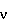) by means of a proportionality constant called Planck's constant (h). The equation is:

```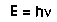(I)
```

We are also told that the speed of light (c) is always equal to the product of the photon's frequency () and wavelength (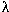):

```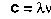(II)
```

In a typical problem, we might be given the energy (E) of a particular photon and asked to find its wavelength (). The speed of light c is always known, so we must find a relationship between what is unknown (the wavelength) and those quantities that are known (energy and speed of light).

In Eq. (I), we must replace, which is unknown. To do this, solve Eq (II) to obtain an expression forin terms ofand c:

```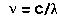(III)                                                                           (                  (found by dividing both sides of (II) by )
```

In Eq. (I), replacewith the above equivalence:

```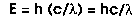(IV)
```

Solve Eq (IV) to isolate the only unknown quantity () on the left side:

```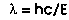(V)
```

(found by multiplying both sides by and dividing both sides by E)

Now substitute in numerical values for h, c, and E into Eq. (V) and you obtain a numerical result for the wavelength .